#### Concept of Impedance and Resistive-Capacitive Impedance in AC Circuits

Impedance:

An ac network usually contains current and voltage sources which are time varying, very generally sinusoidal. The circuit elements might be resistive, capacitive or inductive and will usually be a combination of such. Consequently, there will be resultant magnitude and phase relationships between currents and voltages at different locations all through the circuit. Consideration will be confined to circuits containing only a single driving source.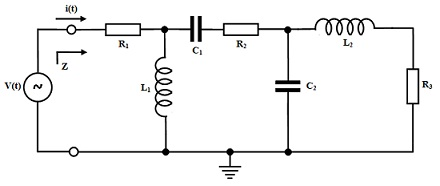Definition of Impedance:

Impedance, Z, is defined as the ratio of voltage difference to current flowing between any two nodes in an ac circuit. Our interest will mainly be in this property as seen by the source driving input terminals of a network. In common, it is complicated in nature, having a real and an imaginary part. The real part is a resistive part whereas the imaginary part is the reactance. Since it is a complex quantity it too has phase and magnitude.

Z = v(t)/i(t) = Real(Z) + Imag(Z) = R ± jX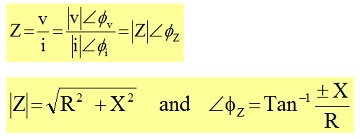Resistive-Capacitive Impedance:

Consider an R-C circuit driven by an ac voltage source as shown below. This comprises resistive and reactive components and therefore must be considered as comprising impedance.

ω = 2 Π f

f = 50 Hz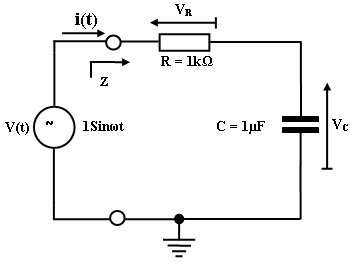Since the elements are in series, the voltage drops across them should sum to the voltage of source driving the circuit and hence:

Z = v(t)/i(t) and v(t) = vR(t) + vC(t)

Then, Z = [vR(t) + vC(t)]/i(t) = [vR(t)/i(t)] + [vC(t)/i(t)] = ZR + ZC

Z = R - jXC = R – j (1/ ωC)

This can be seen that the overall impedance of the network is provided by the impedances of an individual element in series. Therefore, these are simply added altogether just as in the situation of purely resistive elements in series.

This is of interest to establish all the current and voltage relationships that apply to this circuit.

V(t) = Vm sin ωt = Vm <00 is considered as the reference phase of zero.

At first:   R = 1KΩ, C = 1μF = 10-6 F

ω = 2 Π f = 2 x 3.14 x 50 = 314 rad/s

XC = 1/ωC = 1/(314 x 10-6) = 106/314 = 3184.7 = 3.18 K Ω

For the circuit given:

Z = R + 1/j ωC = R – j(1/j ωC)

Z = 1KΩ - j3.18 KΩ = 103 – j3.18 x 103 ?

In terms of magnitude and phase:

|Z| = √106 + (3.18)2 x 106 = √11.1 x 106 = 3.33 KΩ

Consider the current i: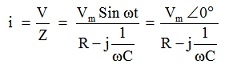Treating Sin ωt as the reference zero phase

Rationalising: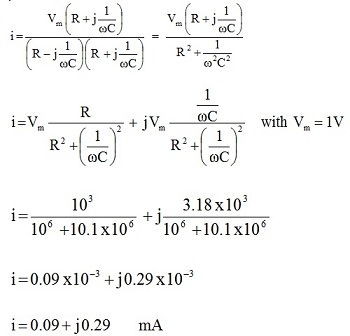Then,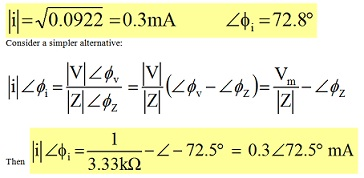This signifies we only require doing one complex number computation. A phasor plot of all currents and voltages concerned is as shown below.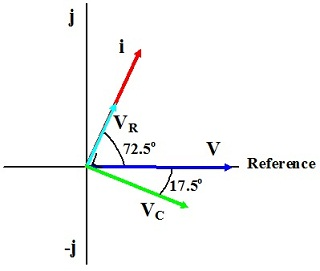When the driving source voltage is taken as reference V , the current leads this by 72.5o as shown by i in the diagram. The voltage across resistor, VR, should have similar phase as the current i as it is purely real. The voltage across capacitor, VC should lag the current via it by 90o and thus lags the source voltage by 17.5o.

Combined Resistive-Inductive Impedance:

Let consider an R-L circuit driven by the ac voltage source as shown below. This also comprises resistive and reactive components and has related impedance.

Ω = 2 Π f

f = 50 Hz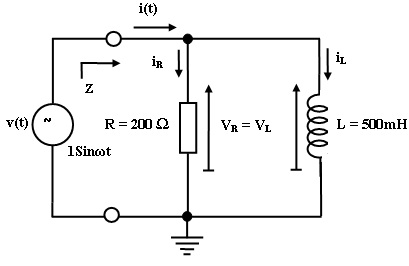It is as well of interest to establish all the current and voltage relationships that apply to this circuit for comparison.

v(t) = Vm sin ωt = Vm <00

taking this as reference.

If, R = 200 Ω, L = 500 mH, Vm = 1V and f = 50 Hz

Then,

ω = 2 Π f = 314 rad/s

XL = ωL = 314 x 500 x 10-3 = 0.5 x 314 = 157 Ω

For the circuit given the inductor and resistor are in parallel and the source voltage emerges across both and hence:

Z = v(t)/i(t) and v(t) = vR(t) = vL(t)

The current drawn from supply is shared among the two elements and hence:

i(t) = iR(t) + iL(t)

Z = v(t)/i(t) = [v(t)]/[iR(t) + iL(t)]

And hence,

1/Z = [iR(t) + iL(t)]/v(t) = [iR(t)/v(t)] + [iL(t)/v(t)] = (1/ZR) + (1/ZL)

1/Z = 1/R + 1/jωL

Where there are just two elements this can be opportunely simplified:

1/Z = (ZL + ZR)/ZRZL

Z = ZRZL/(ZL + ZR) = (j ωLR)/(R + jωL)

Rationalising: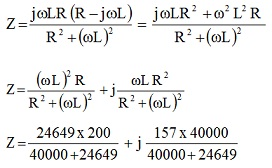Z = 76.25 + j 97.14 Ω

In terms of phase and magnitude:

|Z| = √5814 + 9436 = 123.5 Ω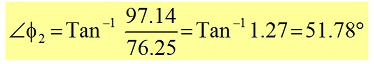To calculate the current drawn from the supply: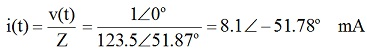The current flowing via the resistor should be in phase with the voltage across it, that is the source voltage, v(t), and thus has a phase of zero.

|iR| = |v(t)|/R = 1<00/R = 1/200 = 5 < 00 mA

The current flowing via the inductor should lag the voltage across it, that again is the source voltage, v(t), and thus this has a phase of -90o.

iL = v(t)/ (ωL L90o) = 1/157 L90o = 6.4 L-90o mA

The phase relationships of all voltages and currents are shown in the phasor diagram below.

V, VL and VR all have similar phase which is that of input source voltage, that is treated as the reference zero phase. The current flowing via the resistor R also has similar zero phase. The current via the inductor lags the voltage across it and thus has a phase of -90o. Ultimately the current drawn from the supply, i, is shown lagging the source voltage by 51.78o as computed.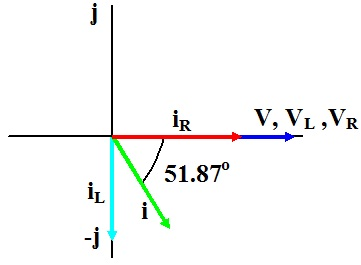Latest technology based Electrical Engineering Online Tutoring Assistance

Tutors, at the www.tutorsglobe.com, take pledge to provide full satisfaction and assurance in Electrical Engineering help via online tutoring. Students are getting 100% satisfaction by online tutors across the globe. Here you can get homework help for Electrical Engineering, project ideas and tutorials. We provide email based Electrical Engineering help. You can join us to ask queries 24x7 with live, experienced and qualified online tutors specialized in Electrical Engineering. Through Online Tutoring, you would be able to complete your homework or assignments at your home. Tutors at the TutorsGlobe are committed to provide the best quality online tutoring assistance for Electrical Engineering Homework help and assignment help services. They use their experience, as they have solved thousands of the Electrical Engineering assignments, which may help you to solve your complex issues of Electrical Engineering. TutorsGlobe assure for the best quality compliance to your homework. Compromise with quality is not in our dictionary. If we feel that we are not able to provide the homework help as per the deadline or given instruction by the student, we refund the money of the student without any delay.